ATD Surface and Sounding Systems Facility | SSSF Observing Facilities Descriptions

## 5.0 Sounding Data Processing

### 5.1 Real Time Data Processing

Sounding data are generated in real time during all soundings, balloonborne or drops from aircraft. These data are available for graphic and tabular display on the sounding system personal computer. The displayed data include horizontal wind components, position, the measured thermodynamic parameters, and parameters calculated from those measured thermodynamic parameters - dew point, geopotential altitude, and ascent rate. In the ISS, the sounding data are also available for display through the ISS Sun workstation using "zeb" display software.

Several data files are generated for each sounding. The various files contain raw thermodynamic and navaid data, ten-second calculated data, calibration information, and housekeeping information. These files are in binary format and are saved for final post processing.

#### 5.1.1 Radiosonde Horizontal Wind and Position Calculation

##### 5.1.1.1 Wind Data Calculation
The horizontal wind components and position are generated by the CLASS system personal computer using data ouput from either the Omega or Loran navigator, depending on the navaid being used. A Trimble TMO model 8402 OMEGA navigator is used to process Omega signals. An Advanced Navigations, Inc. (ANI) model 7000 navigator is used with Loran signals.

In Omega windfinding, Omega phase difference data are sent via RS-232 to the onboard personal computer where they are converted to range data (distance from Omega source) for calculation of both wind and position. A least squares quadratic (second order) polynomial fit is made to the navaid range data from each station over a 240 second smoothing interval. The resulting coefficient of the first order term is taken as an estimate of the slope of the radionavigational data (time derivative of the least squares fit to the individual station data). The slope estimate and the variance of each station's data are used by the wind algorithm to compute the wind components and position. Each station's data are weighted by the inverse of the variance for that station so that the noisiest data are weighted the least.

The horizontal wind components and their variances are computed every ten seconds using 240 seconds of Omega data. The algorithm used is similar to that in Passi and Morel (1987). The ten-second wind and position data are generated with a "centered sliding window" smoothing technique, i.e. each ten-second value is generated from navaid range data taken over the interval from 120 seconds before until 120 seconds after that particular point. The longer sampling period used with Omega is required because of the high level of noise encountered in measurement of the Omega phase. High phase jitter requires increased smoothing. Omega stations transmit for a period of one second every ten seconds. Thus, a sampling interval of 240 seconds gives roughly 24 samples from which to calculate the winds.

In the data processing used with Omega windfinding, the first 120 seconds of wind data (one-half of the required smoothing interval) in any sounding are obtained by interpolation between the wind value taken from the surface meteorological instrumentation at launch and the first ten-second wind datum that is obtained using 240 seconds of navaid data (i.e. the wind datum obtained at ~ 130 seconds after launch).

In Loran-C windfinding, the data output by the ANI 7000 are sent via RS-232 to the personal computer where horizontal wind data are calculated from the Loran station TOA information. A least squares quadratic (second order) polynomial fit is made to the TOA data from each station over a 60 second smoothing interval. As in Omega windfinding, the resulting coefficient of the first order term is taken as an estimate of the slope of the radionavigational data (time derivative of the least squares fit to the individual station data). The slope estimate and the variance of each station's data are used by the wind algorithm to compute the wind components and position. Each station's data are weighted by the inverse of the variance for that station so that the noisiest data are weighted the least.

The horizontal wind components and their variances are computed every ten seconds using 60 seconds of Loran TOA information. Again, the algorithm used is similar to that in Passi and Morel (1987). Roughly 20 sets of TOA data from each station are used in the calculation of each ten-second wind datum (the ANI sampling interval is roughly 3 seconds). The ten-second wind data are generated with a "centered sliding window" smoothing technique, i.e. each ten-second value is generated from TOA data ranging from 30 seconds before until 30 seconds after any given ten-second data point.

##### 5.1.1.2 Position Calculation
When the Omega navaid is used, position is determined by integration of the wind data through the sounding starting with the known launch position (the known site position or the aircraft position at launch in the case of a dropsonde). This is not the case with the Loran navaid. Position is obtained directly from the ANI 7000 if that position is acceptable according to constraints imposed by the navigator itself. If the Loran position is not acceptable the position is obtained from integration of the winds (calculated independently of the position value) from the last good position value.

The Loran position determination scheme can lead to noticeable jumps in the position data when shifting from one mode to the other. Jumps can also occur as Loran stations are either lost or gained by the navigator and the "acceptable" position becomes better or worse. A position deemed acceptable obtained from three Loran stations may be markedly improved as a fourth Loran station is acquired. Such an occurrence can also produce a jump in the position data. Similarly, a jump in "acceptable" position data can occur as a Loran station is lost.

#### 5.1.2 Radiosonde Thermodynamic Data Handling

In the balloonborne soundings using the Vaisala radiosonde, thermodynamic data are received from the sonde in an asynchronous fashion, with respect to the navigation data, as a transmitted analog tone at a data rate of close to one every 1.5 seconds. These data are decoded by the thermodynamic data processor and passed on to the sounding system personal computer.

The raw thermodynamic data are smoothed over a pre-defined interval using a linear least squares technique. The least squares fit is used to generate ten-second thermodynamic data which is used in subsequent data products. The resulting ten-second thermodynamic data are merged with the ten-second wind data and both are then available for display.

In the dropsonde application, thermodynamic data are received from the sonde in an asynchronous fashion, with respect to the navigation data, in a digitized data packet four times a second. They are merged with the ten-second wind data and both are available for display on the aircraft. The merged ten-second thermodynamic (PTH) data are obtained from the asynchronous data stream by interpolating between the first good data points found before and after the ten-second data point desired.

The dropsonde PTH data are time tagged and also contain a telemetry check. If the telemetry check is not zero and set to 255 the data point is considered bad. The telemetry check is obtained from CRC (cyclic redundancy check) error code in each data frame. The details of the CRC code and telemetry check are beyond the scope of this presentation. (See discussion of dropsonde PTH data in the following sections.)

The thermodynamic data measured by the sonde are pressure, temperature, and humidity. These parameters are subsequently used to calculate other parameters - dew point, geopotential altitude, and ascent rate. The algorithms used to calculate those parameters are discussed in following sections.

### 5.2 Sounding Data Post Processing

The post processing step incorporates all the processing that is available in real time processing and allows for "fine tuning" of that real time processing. All the algorithms used in the post processing are identical to those used in real time. The winds are calculated in the same fashion, as are the other derived parameters.

The binary data files created by the data system are saved for use in post processing to produce the final sounding data set. That data set is generated from the binary data files in one of two methods. The binary data are either directly re-formatted or they are re-processed to generate the final data set.

Three different ASCII data files are produced for each individual sounding in the final data set. Each file has a header section identifying the data file type as, "Raw Thermodynamic Data", "Ten-Second Data", or "Interpolated Data". The header section also provides information regarding the particular sounding and its processing. The headers for each data file type are identical except for the data type designation identified above and the data column headers in the case of the "Raw Thermodynamic Data" file. Examples of the three types of data files, including headers, are presented in the appendices.

The filenames of these three files are identical with the exception of the first character. The "Raw Thermodynamic Data" filename first character is `m'. The "Ten-Second Data" filename first character is `x'. The "Interpolated Data" filename first character is `i'. That character is then followed by the month, day, and time in the format mddhhmm, and .xxx, where xxx is a project identifier, usually referencing the site (e.g. i2082300.nau is the "Interpolated Data" file from the sounding made at Nauru at 2300 on the 8th of February). The designators for the month in the filename convention are 1-9, a, b, and c. The numbers 1-9 correspond to January through September, a corresponds to October, b corresponds to November, and c corresponds to December.

Both the "Raw Thermodynamic Data" file and the "Ten- Second Data" file are essentially raw data files containing information calculated in real time. Little attempt is made to edit or perform quality control on these two data files. The "Interpolated Data" file on the other hand is subject to data quality control. The data in this third file are generated from data in the "Ten-Second Data" file which have passed certain data quality constraints (see Table 16).

#### 5.2.1 Raw Thermodynamic Data

##### 5.2.1.1 Balloonborne Soundings
The "Raw Thermodynamic Data" file contains thermodynamic data at a rate of roughly one sample every 1.5 seconds. The data include time in seconds, pressure (mb), temperature (C), dew point (C), relative humidity (%), and an error flag.The values for pressure, temperature, and relative humidity are calibrated values of measurements made directly by the sonde and transmitted to the surface sounding site. Calibration coefficients are applied to the transmitted sonde data by the NCAR RS80 "Met" processor in the sounding data system. The dew point values are calculated from the measured relative humidity according to the equations presented in section 5.3.

The error flag is not used in the balloonborne radiosonde application, it is only used with the dropsonde application. That error flag is set to 777 in the "Raw Thermodynamic Data" files produced from balloonborne soundings.

##### 5.2.1.2 Dropsondes
The "Raw Thermodynamic Data" file contains four samples per second of asynchronous thermodynamic data. That data include time in seconds, pressure (mb), temperature (C), dew point (C), relative humidity (%), and an error flag.The values for pressure, temperature, and relative humidity are calibrated values of measurements made directly by the sonde and transmitted to the aircraft. The dew point value is calculated from the measured relative humidity, according to the equations presented.

The error flag is set based on whether there is a telemetry error or not. If there is an error, the flag is set to 255. The flag is 0 if there is no telemetry error. If the flag is set to 255, the data point is not used in any subsequent data handling (not incorporated into the 10 second data file). The telemetry is monitored using a CRC (cyclic redundancy check) error code in the transmitted data frame. The details of the CRC processing will not be included in this report. The error flag is set to either 0 or 255 automatically. It can be manually adjusted in post processing.

One thing required in dropsonde post processing is to establish a "splash point", the point where the sounding ends, for each drop. In post processing splash point determination, the error code will be altered for that splash point. Remember that the hardware sets only two values for the error code, either 0 for a good data frame or 255 for a bad data frame. If the person doing the processing selects a good data frame as the splash point, the error code is set to 256 by that person. If the person decides that the splash point occurred at a data point tagged 255 and that data point has a good pressure (very possible as the CRC code will set the error code to 255 for any part of the data frame being bad or missing), other values become possible for the error code at the splash point.

The bits for good or bad pressure, temperature, and humidity are the three least significant bits. Bit 0 is the pressure bit, bit 1 is the temperature bit, and bit 2 is the humidity bit. (These can be set or unset by the person doing the processing.) Thus if the only requirement for a splash point is that the pressure is good, the splash point could be any of the following values: 256, 510 (256+254), 508 (256+252),506 (256+250), 504 (256+248). Table 15 shows the error code bit patterns. Only 0 and 255 are set by the hardware, the others are set by human interaction.

```TABLE  15  Bit Maps of Error Code Values
----------------------------------------
Value  - - - - - - - S  - - - - - H T P
----------------------------------------
0      0 0 0 0 0 0 0 0  0 0 0 0 0 0 0 0
255    0 0 0 0 0 0 0 0  1 1 1 1 1 1 1 1
256    0 0 0 0 0 0 0 1  0 0 0 0 0 0 0 0
248    0 0 0 0 0 0 0 0  1 1 1 1 1 0 0 0
249    0 0 0 0 0 0 0 0  1 1 1 1 1 0 0 1
250    0 0 0 0 0 0 0 0  1 1 1 1 1 0 1 0
251    0 0 0 0 0 0 0 0  1 1 1 1 1 0 1 1
252    0 0 0 0 0 0 0 0  1 1 1 1 1 1 0 0
253    0 0 0 0 0 0 0 0  1 1 1 1 1 1 0 1
254    0 0 0 0 0 0 0 0  1 1 1 1 1 1 1 0
----------------------------------------
```

#### 5.2.2 Ten-Second Data

The "Ten-Second Data" file contains thermodynamic data, horizontal wind data, position data, and quality information for the thermodynamic and horizontal wind data. The data are calculated every ten seconds. As in the "Raw Thermodynamic Data" file, the variables pressure, temperature, and relative humidity are calibrated values from measurements made by the sonde. The dew point is calculated from the relative humidity. The "Ten-Second Data" file is meant to be a "raw" data file, that is, it contains data calculated as received with little or no editing or quality control applied to it.

In the balloonborne application, the ten-second thermodynamic data, pressure, temperature, relative humidity and dew point are generated by smoothing the raw thermodynamic data over a pre-defined smoothing interval using a linear least squares technique. The smoothing interval, centered on the ten-second data point, is either 10 or 20 seconds, depending on the sounding system "Met" processor used (10 seconds with the NCAR RS80 "Met" processor). The processor used and the smoothing interval are listed in the data file header.

In the dropsonde application, the ten-second thermodynamic data, pressure, temperature, relative humidity and dew point are obtained by interpolation across the desired ten-second data point, using data from the "Raw Thermodynamic Data" file. The first good thermodynamic data points (pressure, temperature, etc.) obtained before and after the desired ten-second time are used to interpolate the value for that particular ten-second data point. A raw thermodynamic data point is not used if the error flag is set to 255. If there are no good thermodynamic data during the 10 seconds prior to or after any particular ten-second data point, that point's thermodynamic data are set to 999.0 with the quality parameter, Q, set to 99.0.

As an example (in the portion of the file x2010236.d8b included in the appendices), the pressure at 10 seconds (350.7 mb) is an interpolated value obtained from the "Raw Thermodynamic Data" values at 9.9 seconds (350.5 mb) and 10.1 seconds (350.8 mb). Similarly the temperature at 10 seconds (-17.1 C) is obtained from interpolation between the same two points in time (-16.9 C and -17.2 C).

NOTE: Do not confuse this interpolation step with the interpolation used to obtain the "evenly spaced (5mb) pressure level data" file described as the "Interpolated Data" in the next section.

The altitude value is calculated from the hydrostatic equation using pressure, temperature, and dew point. The rate of ascent or descent is obtained from the altitude difference between two successive time steps. The position (lat, lon), angle and range come from the navaid and altitude data.

All wind data - Uwind, Vwind, Wspd, Dir, Qu, Qv, and Quv - are computed from radionavigation data received from the sonde. The wind algorithm returns both wind data (u-component, v-component, windspeed, and wind direction) and the variance and standard deviation of the estimates of that wind data (except in the case of wind direction). The standard deviation returned by the wind algorithm is the quality parameter or Q value for a given wind component, and is an indicator of the noise or quality of the measurement. A lower number reflects higher quality.

The quality parameters are: Qp for pressure, Qt for temperature, Qh for humidity, Qu for the easterly wind component, Qv for the northerly wind component, and Quv for the horizontal windspeed. The quality parameters are the square roots of the variances of the parameter estimates generated from the smoothing intervals used for their calculation. The units of the quality parameters are the same as those for the parameters measured.

Quality parameters are only calculated for the thermodynamic data, pressure, temperature, and relative humidity, in the balloonborne sounding application. In the dropsonde application there is no smoothing interval for pressure, temperature, and relative humidity and thus there are no variance and standard deviation generated. (The ten-second thermodynamic values are obtained by interpolation across two points on either side of the desired point. The smoothing technique is not required, as the integrity of the telemetry link of the digital sonde is known (CRC error code) and any measurement uncertainty due to noise in the transmission is eliminated. Thus, in the dropsonde case, the Qp, Qt, and Qh values are all set to 77.0 which is an indicator of "good data quality" with no noise estimate.)

In the "Ten-Second Data" file, a Q value of 99.0 is used as a bad or missing data flag. Missing data values are set to 999.0. In cases where there are missing data, the gap will be filled by interpolating from existing points. When this happens the Q values are set to 88.0 if the interpolation interval isn't too large. If it becomes too large, the Q value is set to 99.0.

The Q values are set to 99.0 if the sample smoothing interval standard deviation is greater than or equal to 50 (a Q value of 50 or more is an unlikely value for a standard deviation as it would indicated extremely poor and unusable data). This is done to avoid confusion between large Q values from the data smoothing interval standard deviations and the flags, 77.0, 88.0, and 99.0.

Note that relatively large values of Q (values greater than the established noise limits of Table 16) are retained in the "Ten-second" data file. This is due to the raw, unaltered nature of this file. However, all Q values in the "Interpolated Data" file will be less than the established noise limits (except the "flag" Q values of 77.0, 88.0, and 99.0) due to the manner in which that file is generated.

In dropwindsonde applications, there is a period just after launch where experience has shown that the relative humidity (dew point) and wind data are bad. The bad thermodynamic data are due to the abrupt temperature change experienced between the aircraft cabin and the outside atmosphere and the shock of the launch itself. The bad wind data result from the rapid deceleration of the sonde just prior to and shortly after parachute deployment. These parameters are set to 999.0 (Q values set to 99.0) for the period just after launch. The length of the period is a function of altitude and has been determined from experience (see Table 6 in Chapter 1.0).

#### 5.2.3 Interpolated Data

The third data file supplied to the user is the "Interpolated Data" file which is an "evenly spaced pressure level data" file. The data are computed at constant pressure increments of 5 mb. This file is built from the "Ten-Second Data" file by interpolation between ten-second data points. That interpolation is subject to several constraints. By application of the following constraints, the data in the "Interpolated Data" file have been automatically subjected to a quality control based upon both the data Q values and the interpolation interval length used.

The interpolated data are calculated from ten-second data that have Q values less than an established noise limit. That established limit is given for the various parameters in the following table. If the ten-second data Q values (standard deviations) are greater than that noise limit, they are not used to generate the data in the "Interpolated Data" file.

When data points are rejected by the noise limit constraints, the interpolation interval used to obtain a given 5mb data point is lengthened. The interpolation interval can now become a constraint for each variable calculated for the "Interpolated Data" file. If the interpolation interval exceeds a certain value, the data calculated are flagged, using the Q value of 88.0. That value indicates that the particular datum is interpolated across a long interval and thus its quality may be questionable. If that interpolation interval is too long, the datum is considered bad. In that case, the Q value is set to 99.0 but the datum retains the value determined by the interpolation. ALWAYS BE CAREFUL TO CHECK "Q" VALUES!

Table 16 lists the interpolation intervals associated with the different parameters. If the interpolation interval is sufficiently short (within listed constraints) the data values and their associated Q values (standard deviations or standard errors) are determined by direct interpolation of the ten-second values. Otherwise, if the interpolation interval exceeds certain limits, the Q value is assigned either 88.0 or 99.0 depending on the interval length as shown in Table 16.

```TABLE  16  Interpolation Constraints
---------------------------------------------------------------
Variable     Noise Limit    Interpolation Interval   Q value
(seconds)
---------------------------------------------------------------
Pressure     1.0 mb         <=100                 std. error
100 <int <= 200    88.0
>200                  99.0
Temperature  1.0 C          <=50                  std. error
50<int<=100        88.0
>100                  99.0
Relative     3.0%           <=50                  std. error
Humidity                    50<int<=100        88.0
>100                  99.0
Wind         2.0 m/s        <=50                  std.error
50<int<=100        88.0
>100                  99.0

---------------------------------------------------------------
```
The first line in the "Interpolated Data" file, denoted by a negative time is the surface meteorological data. These data are taken from the surface sensors, or aircraft data system in the case of dropsondes, and not from the sonde. This is the case with the "Ten-second Data" file as well. The Q values in this case are set to 77.0 which is to indicate "good data" with no error estimate.

### 5.3 Data Processing Algorithms

#### 5.3.1 Calculation of Dew Point from Relative Humidity

The sonde measures the relative humidity directly. The dew point is then obtained from the measured relative humidity coupled with an expression for vapor pressure. The vapor pressure function used is found in Bolton (1980). It is a good approximation to the results from the generally accepted, but computationally excessive, Goff and Gratch (1946) formulation for vapor pressure.

The computation of dew point temperature follows:

(EQ 1)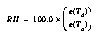so

(EQ 2)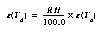Here e(Ta) is saturation vapor pressure, a function of ambient temperature, and e(Td) is ambient vapor pressure, a function of dew point temperature. Both relative humidity and ambient temperature are measured. With an expression for vapor pressure, e(T), the only unknown is dew point temperature, Td.

The vapor pressure function is,

(EQ 3)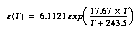where T (degrees Celsius) can be either ambient temperature giving saturation vapor pressure, or dew point temperature giving ambient vapor pressure. Solving the above equations for dew point temperature, Td, yields:

(EQ 4)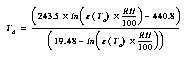#### 5.3.2 Calculation of Altitude Using the Hydrostatic Equation

The altitude is calculated from the hydrostatic equation and the gas law. The calculation uses the average virtual temperature of a layer over which the change in pressure is measured to obtain a layer thickness or change in height. The height change is calculated between each consecutive set of ten-second levels or points. In order to calculate the altitude, the site elevation (msl) or aircraft altitude (msl) must be known.

The computation of altitude follows:

The hydrostatic equation is given by:

(EQ 5)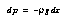where,

• dp is the pressure change over the vertical distance dz,
• r is the density of dry air.
• and, g is the acceleration due to gravity.
Rearranging and using the gas law, gives:

(EQ 6)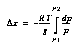where,

• Dz is the change in altitude in meters.
• R is the gas constant for dry air.
• T is the mean temperature for the layer, in degrees K.
• P2 and P1 are the pressures at the top and bottom of the layer, respectively.
Integrating equation 6 and using the mean layer virtual temperature yields:

(EQ 7)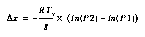where,

• Tv is the mean virtual temperature of the layer, in degrees K.
The mean virtual temperature for the layer, Tv, is calculated from the mixing ratio which is obtained from the mean layer temperature and humidity. The virtual temperature calculation is as follows

(EQ 8)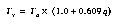:
where,

• Ta is the mean temperature of the layer in degrees K.
• and, q is the specific humidity.
The specific humidity can be expressed in terms of mixing ratio, W, as follows:

(EQ 9)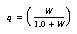Using the approximation, for a small number e:

(EQ 10)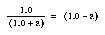Gives:

(EQ 11)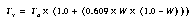where,

• W, the mixing ratio of the layer, is given by:
(EQ 12)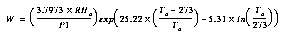where,

• RHa is the mean layer relative humidity.
• P1 is the pressure measured at the current level.
This formulation for mixing ratio is obtained from the definitions of mixing ratio and relative humidity:

(EQ 13)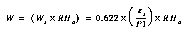where,

• Ws is the saturation mixing ratio.
• es is the saturation vapor pressure.
In equation 12, the formulation used to calculate saturation vapor pressure is taken from page 343 of the "Handbook of Meteorology" (1945). This is given below.

(EQ 14)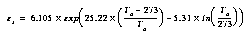The value Dz, obtained from the hydrostatic equation (equation 7), is added (subtracted in the dropsonde case) to the previous level (ten-second data point) altitude value to give the current level (ten-second data point) altitude. This sequential calculation starts from the known station elevation or known aircraft flight level (from radar altimeter).

NOTE: In the layer altitude (thickness) calculation, the mean layer virtual temperature is only used if there is a good humidity as indicated by the humidity quality parameter, Qh. (See discussion of Q values in the next Chapter.). Thus if the humidity quality parameter, Qh, indicates bad humidity data, the mean layer ambient temperature is substituted for the mean layer virtual temperature.

#### 5.3.3 Ascent/Descent Rate Calculation

The ascent/descent rate for each ten-second data point is obtained from the change in altitude calculated above. That ascent/descent rate is simply the calculated altitude change divided by 10, the number of seconds over which the altitude change occurred